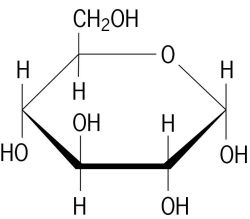Why is respiration an exothermic process?

Feb 16, 2014

Respiration is an exothermic process because it forms the highly stable $\text{C=O}$ bonds of ${\text{CO}}_{2}$.

Explanation:

During respiration, glucose molecules are converted to other molecules in a series of steps.

They finally end up as carbon dioxide and water.

The overall reaction is

$\text{C"_6"H"_12"O"_6 + "6O"_2 → "6CO"_2 + "6H"_2"O" + "2805 kJ}$The reaction is exothermic because the $\text{C=O}$ and $\text{O-H}$ bonds in the products are so much more stable than the bonds in the reactants.

Bond energy is the average energy needed to break a bond.

Some bond energies are:

$\text{C-C}$ = 347 kJ/mol
$\text{C-H}$ = 413 kJ/mol;
$\text{C-O}$ = 358 kJ/mol;
$\text{O-H}$ = 467 kJ/mol;
$\text{O=O}$ = 495 kJ/mol;
$\text{C=O}$ = 799 kJ/mol

We can view the process as breaking all the bonds in the reactants to separate the atoms and then re-combining the atoms to form the bonds in the products.

A glucose molecule has the formulaIt contains 5 $\text{C-C}$, 7 $\text{C-H}$; 7 $\text{C-O}$, and 5 $\text{O-H}$ bonds.

The 6 ${\text{O}}_{2}$ molecules contain 6 $\text{O=O}$ bonds.

The products contain 12 $\text{C=O}$ and 12 $\text{O-H}$ bonds.

The overall process is

$\text{5C-C"color(white)(l) + "7C-H" + "7C-O" +color(red)(cancel(color(black)("5O-H"))) + "6O=O" → "12C=O" + stackrelcolor(blue)(7)(color(red)(cancel(color(black)(12))))"O-H}$

or

$\underbrace{\text{5C-C"color(white)(l) + "7C-H" + "7C-O"+ "6O=O")_color(red)("break these bonds")→ underbrace("12C=O"color(white)(l) + "7O-H")_color(red)("form these bonds}}$

The energy differences in kilojoules per mole are

$\textcolor{w h i t e}{l} \text{5C-C" +color(white)(l) "7C-H" + "7C-O"color(white)(l)+color(white)(l) "6O=O"color(white)(l) → "12C=O" +color(white)(l) "5O-H}$
$\text{5×347" + "7×413" + "7×358"+color(white)(l) "6×495"color(white)(l) → "12×799" + "7×467}$
color(white)(l)1735color(white)(l) +color(white)(l)2891color(white)(l) +color(white)(l) 2506color(white)(l) +color(white)(ll) 2970 color(white)(m)→ color(white)(m)9588 color(white)(ll)+color(white)(l) 3269
$\textcolor{w h i t e}{m m m m m m m} \text{10 102"color(white)(mmmmmmml) → color(white)(m) color(white)(mm)"12 857}$

ΔH ≈ "(10 102 - 12 857) kJ/mol" = "-2755 kJ/mol"

Almost 75 % of the energy released comes from formation of the stable $\text{C=O}$ bonds in ${\text{CO}}_{2}$.